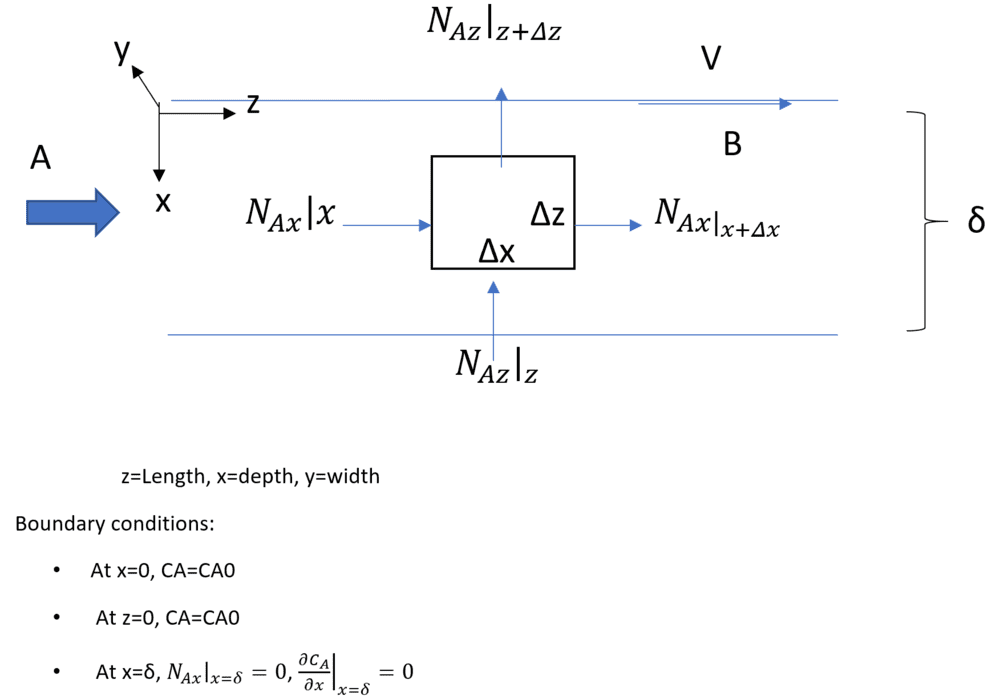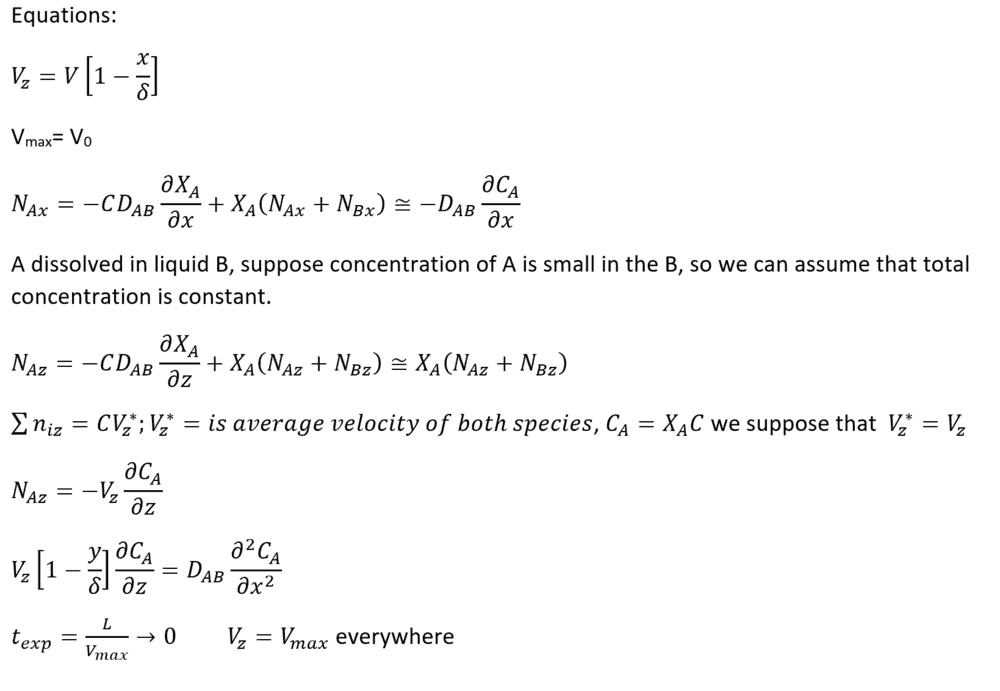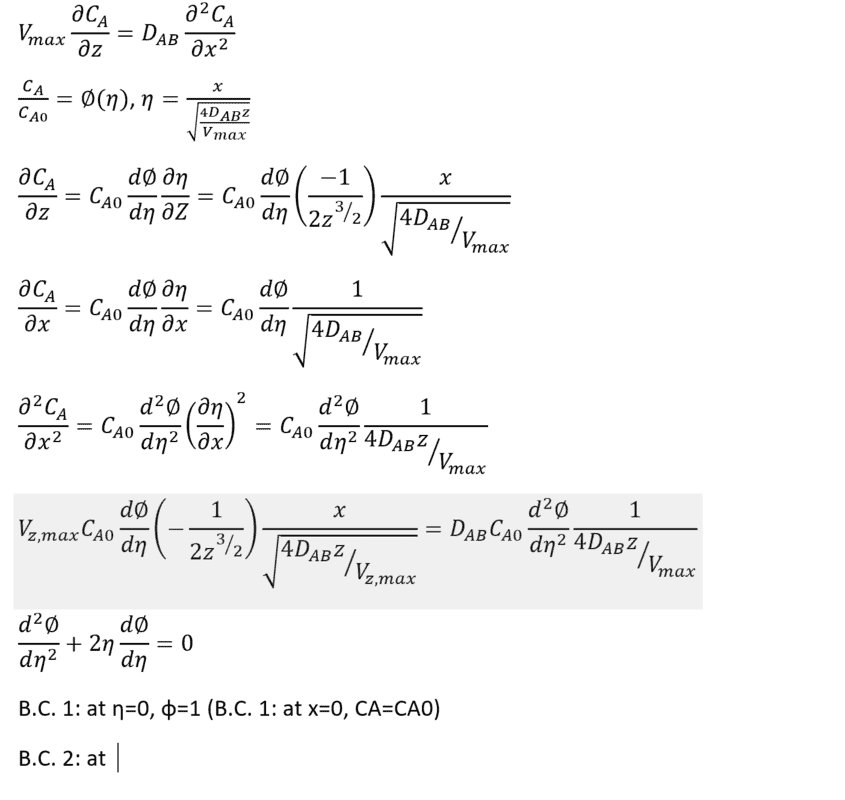Transport phenomena-mass transfer

Hello everyone,

I would like to obtain the equation for mass transfer of contaminant in a river. Here the fluid flow is laminar and I don't have reaction. I solved it and obtained this equation, but I think this equation is wrong because when I solved it numerically I got wrong answers. Would you please help?
Thanks a lot

Attachments

• equation 2.docx
53.7 KB · Views: 275

anorlunda
Staff Emeritus
You might get more and better answers if you post the information directly in the post. I for one will not open a DOCX file from a stranger because of the security risk. PDF is slightly better, but still a problem.

You can copy/paste text, and even screen shots into a post. Just make sure the photos are easy to read on any screen, including phones.

You might get more and better answers if you post the information directly in the post. I for one will not open a DOCX file from a stranger because of the security risk. PDF is slightly better, but still a problem.

You can copy/paste text, and even screen shots into a post. Just make sure the photos are easy to read on any screen, including phones.
Thank you for comment

You might get more and better answers if you post the information directly in the post. I for one will not open a DOCX file from a stranger because of the security risk. PDF is slightly better, but still a problem.

You can copy/paste text, and even screen shots into a post. Just make sure the photos are easy to read on any screen, including phones.
Thank you for comment. Here is screen shots: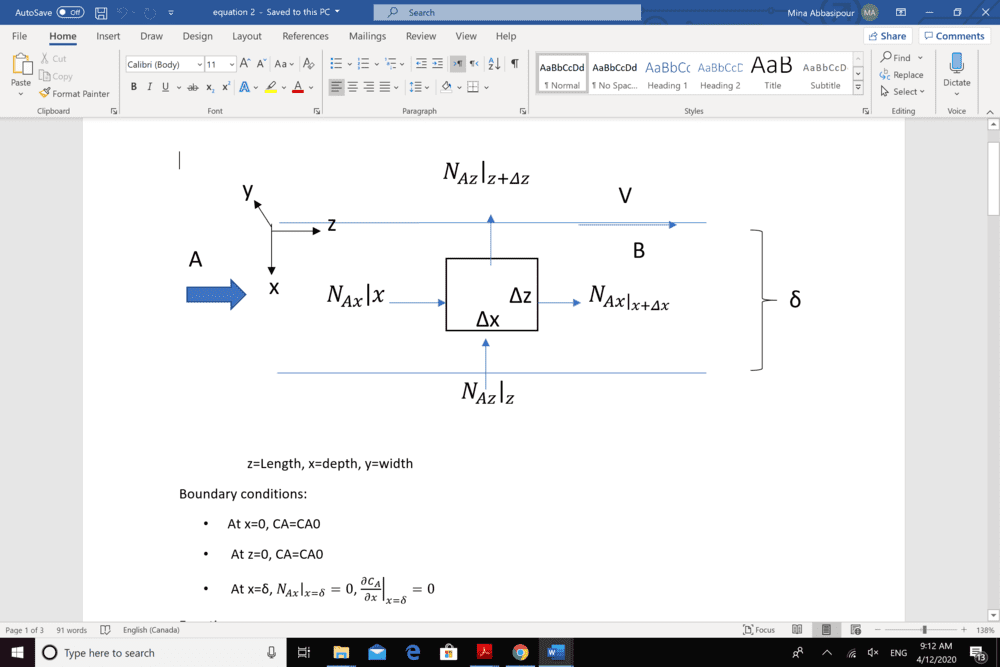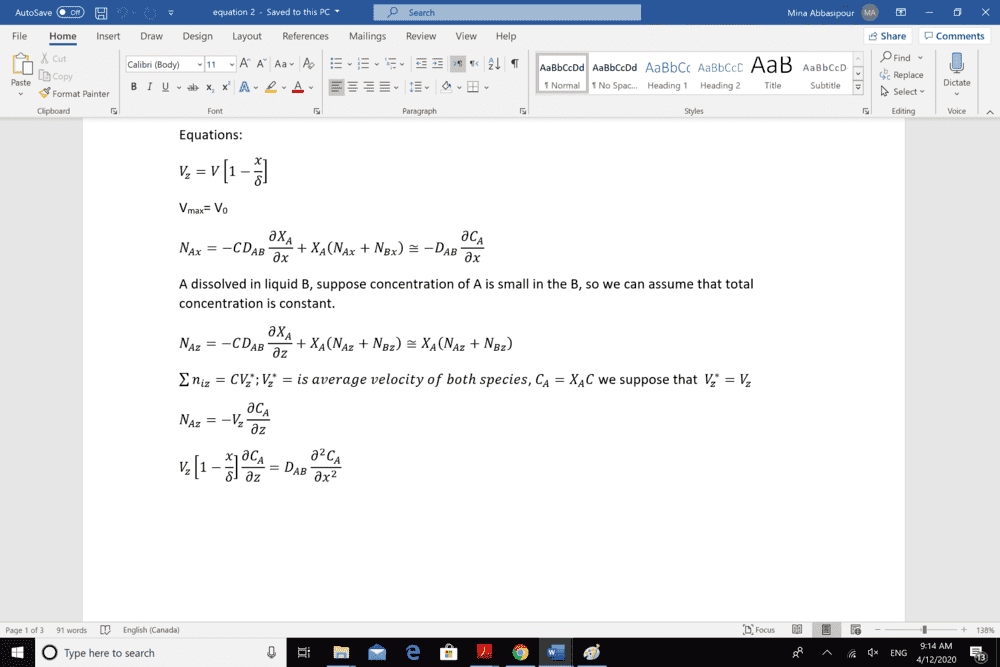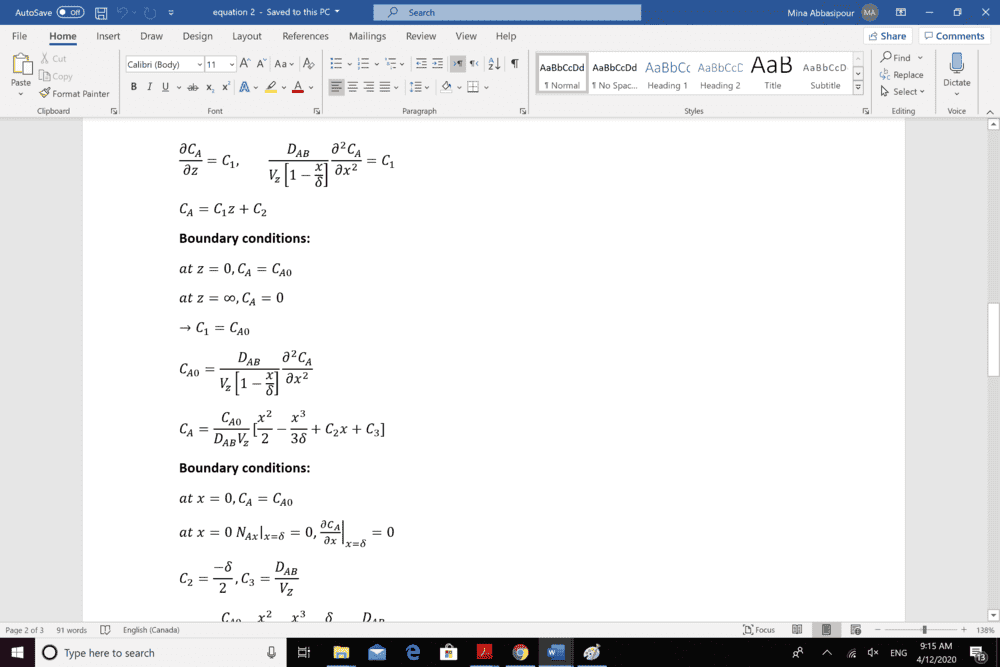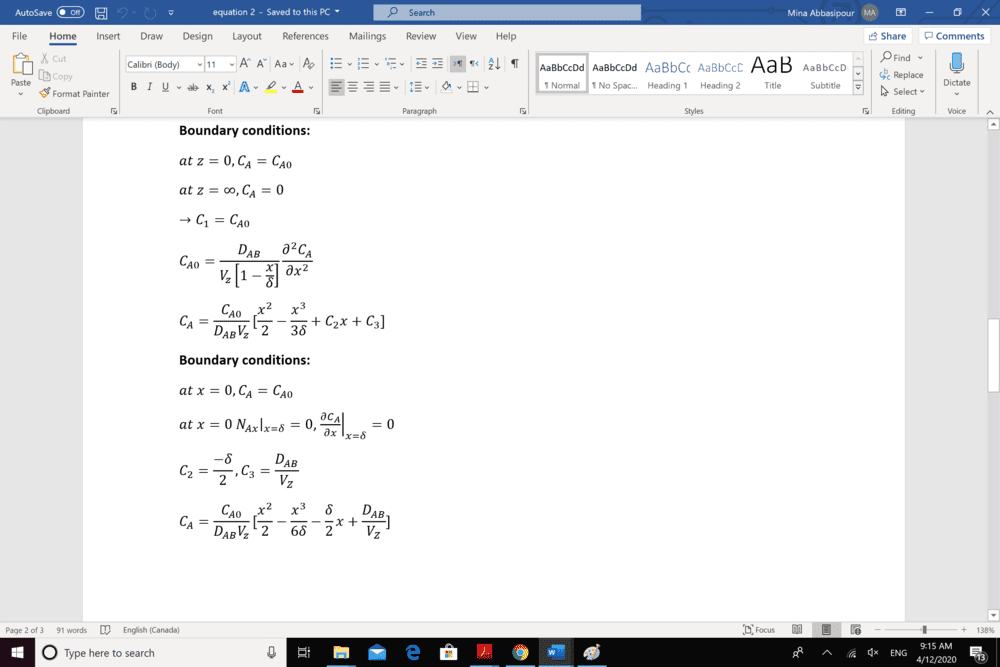•anorlunda
Thank you so much for your reply. I try to obtain mass transfer of contaminants in a river. I suppose that my problem is laminar flow and I don't have reaction. I suppose that (A= contaminate particles, B=water)
X= depth, Z= length and Y= width of river
Boundary conditions: at x=0 , Z=0 CA=CA0 and at Z=delta (depth of river) dCA/dx=0
Vz= V(1-x/delta)
Vz(1-x/delta)dCA/dz= -DAB (d^2CA/dx^2)
I don't know my way is correct or not and if it is correct how should I continue it?

Thanks

Chestermiller
Mentor
I see the equations you are trying to solve and understand the physical problem you are trying to solve. Your formulation looks OK to me. But your solution method to this set of partial differential equations in x and z (as presented in the screen shots) is totally incorrect. You also alluded to a numerical solution. Is that different from what is shown in the screen shots? The solution to the partial differential equation and boundary conditions you formulated is ##C(x,z)=C_{A0}##.

•anni
I see the equations you are trying to solve and understand the physical problem you are trying to solve. Your formulation looks OK to me. But your solution method to this set of partial differential equations in x and z (as presented in the screen shots) is totally incorrect. You also alluded to a numerical solution. Is that different from what is shown in the screen shots?
Thank you so much for your reply. are the boundary conditions correct or not?

Today I solved them in another way. I suppose that Vz=Vz, max and solved the equation. But now the obtained results were completely different with Comsol simulation. I don't know where is my problem.

Chestermiller
Mentor
Maybe you want the BC at z = 0 to be C = 0?

I supposed that where the contaminant is pouring in the river is X=0 and Z=0

Chestermiller
Mentor
I supposed that where the contaminant is pouring in the river is X=0 and Z=0
Then in your steady state formulation, it is going to propagate downstream and fill the entire region. Check it out. Substitute ##C(x,z)=C_{A0}## in your differential equation and BCs. You will find that it satisfies them exactly. And, since the equations are linear, it is the unique solution.

•anni
Then in your steady state formulation, it is going to propagate downstream and fill the entire region. Check it out. Substitute ##C(x,z)=C_{A0}## in your differential equation and BCs. You will find that it satisfies them exactly. And, since the equations are linear, it is the unique solution.
I am not if I understood what you mean. Do you mean the equation and my BCs are correct?

Chestermiller
Mentor
I am not if I understood what you mean. Do you mean the equation and my BCs are correct?
What I am saying is that the solution to your equations as formulates is ##C(x,z)=C_{A0}##. Your differential equation is OK, but there is a problem with how you are specifying the boundary conditions. The correct formulation depends on the precise details of what is happening at the boundaries in the actual physical system. The boundary conditions you have specified does not capture the essence of what is happening at the boundaries in the actual physical system you are trying to describe.

•anni
Thank you so much for your explanations. So, what Bcs should I specified to obtain correct result?

Chestermiller
Mentor
Thank you so much for your explanations. So, what Bcs should I specified to obtain correct result?
As I said, it depends on the details of the actual physical system. Also, maybe you want to be looking at the transient behavior of the system, rather then the steady state behavior?

I gotta go now. Be back later.

In real system it is not steady state and it is turbulent. But I can solve it simply, I suppose that it is laminar and steady state.

Chestermiller
Mentor
The thing that is causing the problem with your analysis is the boundary condition at z = 0. As long as it is ##C_{A0}##, it is going to be that value throughout the solution domain at steady state. You just can't have the entire stream flowing into the region at that concentration. Try the calculation with this value set to zero, just to see how the solution comes out.

•anni
The thing that is causing the problem with your analysis is the boundary condition at z = 0. As long as it is ##C_{A0}##, it is going to be that value throughout the solution domain at steady state. You just can't have the entire stream flowing into the region at that concentration. Try the calculation with this value set to zero, just to see how the solution comes out.
[/QUO
Thank you so much

•Chestermiller
I solved the problem again but I don't know what should I do for next step.